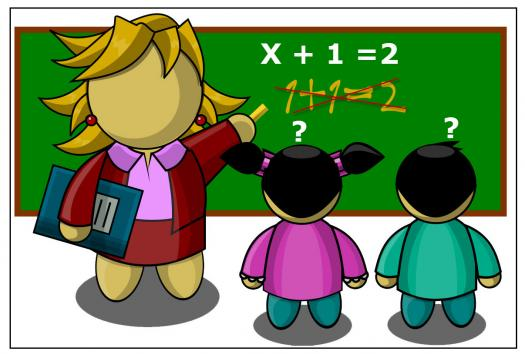# Can You Pass This Algebra Test For Kids?

Approved & Edited by ProProfs Editorial Team
At ProProfs Quizzes, our dedicated in-house team of experts takes pride in their work. With a sharp eye for detail, they meticulously review each quiz. This ensures that every quiz, taken by over 100 million users, meets our standards of accuracy, clarity, and engagement.
| Written by Anouchka
A
Anouchka
Community Contributor
Quizzes Created: 660 | Total Attempts: 453,833
Questions: 10 | Attempts: 315SettingsOur kids don't really like algebra. And it doesn't even matter if it's when they reach high school or not, they truly hate it. The funny thing is that algebra for kids is quite simple and very easy. Parents actually enjoy seeing their kids solve algebra problems because they see them as future geniuses. Most of the time, they are not wrong to think so. Have your kid complete our small algebra quiz for fun.

• 1.

### What's the double of 5?

• A.

10

• B.

2

• C.

12

• D.

5

A. 10
Explanation
The question asks for the double of 5, which means multiplying 5 by 2. The correct answer is 10 because 5 multiplied by 2 equals 10.

Rate this question:

• 2.

### What result do you find when you add the number 11 three times?

• A.

20

• B.

21

• C.

111

• D.

33

D. 33
Explanation
When you add the number 11 three times, you get the result of 33. This is because 11 + 11 + 11 equals 33.

Rate this question:

• 3.

### How do you simplify 2+2+6?

• A.

5+5

• B.

2(2+6)

• C.

6+2

• D.

2+ 6

A. 5+5
Explanation
The correct answer is 5+5 because when you simplify 2+2+6, you add the numbers together to get 10. Therefore, 5+5 is the simplified form of the expression.

Rate this question:

• 4.

### Which number is a multiple of 10?

• A.

55

• B.

2

• C.

11

• D.

100

D. 100
Explanation
100 is a multiple of 10 because it can be evenly divided by 10. When 100 is divided by 10, the result is 10 with no remainder.

Rate this question:

• 5.

### How many times do you find 10 in 1000?

• A.

100 times

• B.

10 times

• C.

20 times

• D.

30 times

A. 100 times
Explanation
In order to find out how many times 10 appears in 1000, we need to divide 1000 by 10. When we divide 1000 by 10, the result is 100. Therefore, 10 appears 100 times in 1000.

Rate this question:

• 6.

### Which number is an even number?

• A.

1

• B.

0

• C.

2

• D.

9

C. 2
Explanation
The number 2 is an even number because it is divisible by 2 without leaving a remainder. Even numbers are those that can be divided evenly by 2, while odd numbers cannot. In this case, 2 divided by 2 equals 1, with no remainder, confirming that it is an even number.

Rate this question:

• 7.

### Which numbers are uneven?

• A.

1,5,9

• B.

2,4

• C.

10,22

• D.

40, 100

A. 1,5,9
Explanation
The numbers 1, 5, and 9 are uneven because they are not divisible by 2 and do not have a remainder of 0 when divided by 2.

Rate this question:

• 8.

### Complete this series: 11-22-33....

• A.

44

• B.

34

• C.

24

• D.

90

A. 44
Explanation
The series follows a pattern where each number is repeated twice. The first number is multiplied by 2 to get the second number, and then the second number is multiplied by 2 to get the third number. This pattern continues throughout the series. Therefore, the next number in the series would be 44, as it is obtained by multiplying 33 (the previous number) by 2.

Rate this question:

• 9.

### How many time do you find 2 in 8?

• A.

Twice

• B.

Four times

• C.

5 times

• D.

7 times

B. Four times
• 10.

### How many times can you find 7 in 35?

• A.

5 times

• B.

6 times

• C.

7 times

• D.

8 timesBack to top